• 多层前馈神经网络有哪些参数_前馈神经网络中的参数数量
千次阅读
2020-09-01 15:33:33

多层前馈神经网络有哪些参数

Machine learning is solving such a large number of sophisticated problems today that it seems like magic. But there isn’t any magic in machine learning rather it has a strong mathematical and statistical foundation.

如今，机器学习正在解决如此众多的复杂问题，似乎就像魔术一样。 但是机器学习没有任何魔力，它具有强大的数学和统计基础。

While trying to understand the important and somewhat difficult concepts of machine learning, we sometimes do not even think about some of the trivial concepts. Maybe you think of those, but I know that I ignore a lot of simple things many a times. The reason is the amazing machine learning and deep learning libraries that have functions and methods to quickly do this for us. 😍

在尝试理解机器学习的重要且有些困难的概念时，我们有时甚至没有考虑一些琐碎的概念。 也许您会想到这些，但是我知道我很多次都忽略了许多简单的事情。 原因是令人惊叹的机器学习和深度学习库，它们具有可为我们快速完成此操作的功能和方法。 😍

One such trivial problem is to find the total number of trainable parameters in a feed-forward neural network by hand. A question that I encountered in one of my exams and confused me with the options provided. This question has also been asked in many different forums by many machine learnimg practitioners. 🙋🏻

一个这样的琐碎问题是用手找到前馈神经网络中可训练参数的总数。 我在一项考试中遇到的一个问题，使我对所提供的选项感到困惑。 许多机器学习实践者也在许多不同的论坛中提出了这个问题。 🙋🏻

The problem discussed in this post is:

这篇文章中讨论的问题是：

# 如何找到前馈神经网络中可训练参数的总数？ (How to find the total number of trainable parameters in a feed-forward neural network?)

You must be wondering why is that even an important one to discuss. It is indeed! The time taken to train a model is dependent on the number of parameters to train so this knowledge can really help us at times.

您一定想知道为什么这甚至是一个重要的话题。 这的确是！ 训练模型所需的时间取决于训练参数的数量，因此这些知识有时确实可以为我们提供帮助。

By looking at a simple network, you can easily count and tell the number of parameters. In the worst case, you can draw the diagram and tell the number of parameters. But what happens when you encounter a question of a neural network with 7 layers and a different number of neurons in each layer, say 8, 10, 12, 15, 15, 12, 6. How would you tell how many parameters are there in all?

通过查看一个简单的网络，您可以轻松地计算并告诉参数数量。 在最坏的情况下，您可以绘制图表并告诉参数数量。 但是，当您遇到一个神经网络的问题，该问题具有7层并且每层中有不同数量的神经元时，比如说8、10、12、15、15、12、6，该怎么办？所有？

Let us together find a mathematical formula to get the count. But before moving to the calculation, let us first understand what a feed-forward neural network is and what characteristics it possesses. This will help us in finding the total number of parameters.

让我们一起找到一个数学公式来获取计数。 但是在进行计算之前，让我们首先了解什么是前馈神经网络以及它具有的特征。 这将帮助我们找到参数的总数。

A feed-forward neural network is the simplest type of artificial neural network where the connections between the perceptrons do not form a cycle.

前馈神经网络是最简单的人工神经网络，其中感知器之间的连接不形成循环。

Despite being the simplest neural network, they are of extreme importance to the machine learning practitioners as they form the basis of many important and advanced applications used today. 🤘

尽管它们是最简单的神经网络，但是它们对于机器学习从业者来说却极为重要，因为它们构成了当今使用的许多重要和高级应用程序的基础。 🤘

Characteristics of a feed-forward neural network:

前馈神经网络的特征：

1. Perceptrons are arranged in layers. The first layer takes in the input and the last layer gives the output. The middle layers are termed as hidden layers as they remain hidden from the external world.

感知器分层排列。 第一层接受输入，最后一层提供输出。 中间层被称为隐藏层，因为它们对外部世界保持隐藏。
2. Each perceptron in a layer is connected to each and every perceptron of the next layer. This is the reason for information flowing constantly from a layer to the next layer and hence the name feed-forward neural network.

一层中的每个感知器都连接到下一层的每个感知器。 这就是信息不断从一层流到下一层的原因，因此也就称为前馈神经网络。
3. There is no connection between the perceptrons of the same layer.

同一层的感知器之间没有连接。
4. There is no backward connection (called a feedback connection) from the current layer to the previous layer.

从当前层到上一层没有反向连接(称为反馈连接)。

Note: A perceptron is the fundamental unit of a neural network that calculates the weighted sum of the input values.

注意：感知器是计算输入值的加权和的神经网络的基本单位。

Mathematically, a feed-forward neural network defines a mapping y = f(x; θ) and learns the value of the parameters θ that helps in finding the best function approximation.

在数学上，前馈神经网络定义了一个映射y = f(x;θ)并学习了有助于寻找最佳函数逼近的参数θ的值。

Note: There is also a bias unit in a feed-forward neural network in all the layers except the output layer. Biases are extremely helpful in successful learning by shifting the activation function to the left or to the right. Confused? 🤔 In simple words, bias is similar to an intercept (constant) in a linear equation of the line, y = mx + c, which helps to fit the prediction line to the data better instead of a line always passing through the origin (0,0) (in case of y = mx).

注意：前馈神经网络中除输出层以外的所有层中还存在一个偏置单元。 偏向通过将激活功能向左或向右移动，对于成功学习非常有帮助。 困惑？ simple简单来说，偏差类似于直线的线性方程y = mx + c中的截距(常数)，这有助于使预测线更适合数据，而不是始终穿过原点的线(0 ，0)(如果y = mx)。

Let us now use this knowledge to find the number of parameters.

现在让我们使用此知识来查找参数的数量。

Scenario 1: A feed-forward neural network with just one hidden layer. Number of units in the input, hidden and output layers are respectively 3, 4 and 2.

方案1：仅具有一个隐藏层的前馈神经网络。 输入，隐藏和输出层中的单位数分别为3、4和2。

Assumptions:

假设：

i = number of neurons in input layer

i =输入层中神经元的数量

h = number of neurons in hidden layer

h =隐藏层中神经元的数量

o = number of neurons in output layer

o =输出层中神经元的数量

From the diagram, we have i = 3, h = 4 and o = 2. Note that the red colored neuron is the bias for that layer. Each bias of a layer is connected to all the neurons in the next layer except the bias of the next layer.

从图中，我们有i = 3， h = 4和o =2。请注意，红色神经元是该层的偏差。 一层的每个偏置都连接到下一层的所有神经元，除了下一层的偏置。

Mathematically:

数学上：

1. Number of connections between the first and second layer: 3 × 4 = 12, which is nothing but the product of i and h.

第一和第二层之间的连接数：3×4 = 12，这不过是ih的乘积。

2. Number of connections between the second and third layer: 4 × 2 = 8, which is nothing but the product of h and o.

第二层和第三层之间的连接数：4×2 = 8，这不过是ho的乘积。

3. There are connections between layers via bias as well. Number of connections between the bias of the first layer and the neurons of the second layer (except bias of the second layer): 1 × 4, which is nothing but h.

层之间也通过偏置连接。 第一层的偏置与第二层的神经元之间的连接数(第二层的偏置除外)：1×4，仅是h

4. Number of connections between the bias of the second layer and the neurons of the third layer: 1 × 2, which is nothing but o.

第二层的偏置与第三层的神经元之间的连接数：1×2，不过是o

Summing up all:

总结所有：

3 × 4 + 4 × 2 + 1 × 4 + 1 × 2

3×4 + 4×2 +1×4 +1×2

= 12 + 8 + 4 + 2

= 12 + 8 + 4 + 2

= 26

= 26

Thus, this feed-forward neural network has 26 connections in all and thus will have 26 trainable parameters.

因此，该前馈神经网络总共具有26个连接，因此将具有26个可训练的参数。

Let us try to generalize using this equation and find a formula.

让我们尝试使用该方程式进行概括并找到一个公式。

3 × 4 + 4 × 2 + 1 × 4 + 1 × 2

3×4 + 4×2 +1×4 +1×2

= 3 × 4 + 4 × 2 + 4 + 2

= 3×4 + 4×2 + 4 + 2

= i × h + h × o + h + o

= i × h + h × o + h + o

Thus, the total number of parameters in a feed-forward neural network with one hidden layer is given by:

因此，具有一个隐藏层的前馈神经网络中的参数总数由下式给出：

(i × h + h × o) + h + o

(i×h + h×o)+ h + o

Since this network is a small network it was also possible to count the connections in the diagram to find the total number. But, what if the number of layers is more? Let us work on one more scenario and see if this formula works or we need an extension to this.

由于该网络是小型网络，因此还可以对图中的连接进行计数以找到总数。 但是，如果层数更多怎么办？ 让我们在另一个场景中工作，看看这个公式是否有效，或者我们需要对此进行扩展。

Scenario 1: A feed-forward neural network with three hidden layers. Number of units in the input, first hidden, second hidden, third hidden and output layers are respectively 3, 5, 6, 4 and 2.

方案1：具有三个隐藏层的前馈神经网络。 输入，第一隐藏，第二隐藏，第三隐藏和输出层中的单元数分别为3、5、6、4和2。

Assumptions:

假设：

i = number of neurons in input layer

i =输入层中神经元的数量

h1 = number of neurons in first hidden layer

h1 =第一隐藏层中的神经元数量

h2 = number of neurons in second hidden layer

h2 =第二个隐藏层中的神经元数量

h3 = number of neurons in third hidden layer

h3 =第三隐藏层中的神经元数量

o = number of neurons in output layer

o =输出层中神经元的数量

1. Number of connections between the first and second layer: 3 × 5 = 15, which is nothing but the product of i and h1.

第一层和第二层之间的连接数：3×5 = 15，这不过是ih1的乘积。

2. Number of connections between the second and third layer: 5 × 6 = 30, which is nothing but the product of h1 and h2.

第二层和第三层之间的连接数：5×6 = 30，仅是h1h2的乘积。

3. Number of connections between the third and fourth layer: 6 × 4 = 24, which is nothing but the product of h2 and h3.

第三层和第四层之间的连接数：6×4 = 24，这不过是h2和h3的乘积。

4. Number of connections between the fourth and fifth layer: 4 × 2= 8, which is nothing but the product of h3 and o.

第四层与第五层之间的连接数：4×2 = 8，这不过是h3o的乘积。

5. Number of connections between the bias of the first layer and the neurons of the second layer (except bias of the second layer): 1 × 5 = 5, which is nothing but h1.

第一层的偏置与第二层的神经元之间的连接数(第二层的偏置除外)：1×5 = 5，仅是h1

6. Number of connections between the bias of the second layer and the neurons of the third layer: 1 × 6 = 6, which is nothing but h2.

第二层的偏置与第三层的神经元之间的连接数：1×6 = 6，这不过是h2

7. Number of connections between the bias of the third layer and the neurons of the fourth layer: 1 × 4 = 4, which is nothing but h3.

第三层的偏置与第四层的神经元之间的连接数：1×4 = 4，这不过是h3

8. Number of connections between the bias of the fourth layer and the neurons of the fifth layer: 1 × 2 = 2, which is nothing but o.

第四层的偏置与第五层的神经元之间的连接数：1×2 = 2，这不过是o

Summing up all:

总结所有：

3 × 5 + 5 × 6 + 6 × 4 + 4 × 2 + 1 × 5 + 1 × 6 + 1 × 4 + 1 × 2

3×5 + 5×6 + 6×4 + 4×2 +1×5 +1×6 +1×4 +1×2

= 15 + 30 + 24 + 8 + 5 + 6 + 4 + 2

= 15 + 30 + 24 + 8 + 5 + 6 + 4 + 2

= 94

= 94

Thus, this feed-forward neural network has 94 connections in all and thus 94 trainable parameters.

因此，该前馈神经网络总共具有94个连接，因此具有94个可训练参数。

Let us try to generalize using this equation and find a formula.

让我们尝试使用该方程式进行概括并找到一个公式。

3 × 5 + 5 × 6 + 6 × 4 + 4 × 2 + 1 × 5 + 1 × 6 + 1 × 4 + 1 × 2

3×5 + 5×6 + 6×4 + 4×2 +1×5 +1×6 +1×4 +1×2

= 3 × 5 + 5 × 6 + 6 × 4 + 4 × 2 + 5 + 6 + 4 + 2

= 3×5 + 5×6 + 6×4 + 4×2 + 5 + 6 + 4 + 2

= i × h1 + h1 × h2 + h2 × h3+ h3 × o + h1 + h2 + h3+ o

= i × h1 + h1 × h2 + h2 × h3 + h3 × o + h1 + h2 + h3 + o

Thus, the total number of parameters in a feed-forward neural network with three hidden layers is given by:

因此，具有三个隐藏层的前馈神经网络中的参数总数由下式给出：

(i × h1 + h1 × h2 + h2 × h3 + h3 × o) + h1 + h2 + h3+ o

(i×h1 + h1×h2 + h2×h3 + h3×o)+ h1 + h2 + h3 + o

## ŤHUS，公式找到可训练参数的总数在具有n个隐藏层的前馈神经网络由下式给出： (Thus, the formula to find the total number of trainable parameters in a feed-forward neural network with n hidden layers is given by:)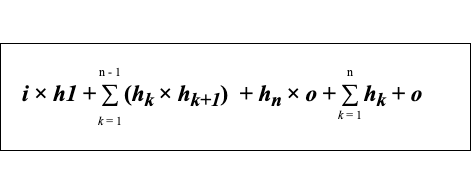Formula to find the total number of parameters in a feed-forward neural network (Image by author)

If this formula sounds a bit overwhelming 😳, don’t worry, there is no need to memorize this formula 🙅. Just keep in mind that in order to find the total number of parameters we need to sum up the following:

如果这个公式听起来有点😳，不要担心，没有必要记住这个公式🙅。 请记住，为了找到参数总数，我们需要总结以下内容：

1. product of the number of neurons in the input layer and first hidden layer

输入层和第一个隐藏层中神经元数量的乘积
2. sum of products of the number of neurons between the two consecutive hidden layers

两个连续的隐藏层之间的神经元数量的乘积之和
3. product of the number of neurons in the last hidden layer and output layer

最后一个隐藏层和输出层中神经元数量的乘积
4. sum of the number of neurons in all the hidden layers and output layer

所有隐藏层和输出层中神经元数量的总和

Now, I hope you can apply this method to find the total number of parameters in a feed-forward neural network with any number of hidden layers and neurons even without using the library. 🙃

现在，我希望您可以使用此方法来查找前馈神经网络中具有任意数量的隐藏层和神经元的参数总数，即使不使用该库也是如此。 🙃

Reference:

参考：

https://cs.stanford.edu/people/eroberts/courses/soco/projects/neural-networks/Architecture/feedforward.html

Thank you! Do leave your comments if you have any feedback or suggestion regarding this post!

谢谢！ 如果您对此帖子有任何反馈或建议，请留下您的评论！

多层前馈神经网络有哪些参数python java 深度学习 人工智能
更多相关内容
• pytorch实现前馈神经网络实验（torch实现）参考链接：https://blog.csdn.net/qq_37534947/article/details/109402126
• 一共13页PPT，概述了线性回归和前馈神经网络，线性回归是利用数理统计中回归分析，来确定两种或两种以上变量间相互依赖的定量关系的一种统计分析方法，运用十分广泛。
• 参考文章：https://blog.csdn.net/qq_37534947/article/details/109394648
• BAT算法工程师深入详细地讲解前馈神经网络，带你轻松入门深度学习!
• 前馈神经网络（反向传播算法）用到的数据集，包含5000张数字图片X及对应标签y。由于是matlab类型的数据，X需要转置。
• matlab开发-快速多层前馈神经网络训练。这是一个简单而快速的代码，可以训练任意层数的神经网络。
• 前馈神经网络是一种最简单的神经网络，各神经元分层排列。每个神经元只与前一层的神经元相连。接收前一层的输出，并输出给下一层．各层间没有反馈。是目前应用最广泛、发展最迅速的人工神经网络之一。研究从20世纪60...
• 此代码使用一阶随机梯度下降优化多层前馈神经网络。 它将网络输出为一个结构，然后可以在新数据上进行测试。matlab
• 使用Python实现简单的前馈神经网络算法。 工作正在进行中。
• 权重系数确定matlab代码使用前馈神经网络和反向传播进行多元分类 该代码已用Matlab（基本版本，没有额外的工具箱）编写，并在8.3版（R2014a）上进行了测试。 所用方程式的主要参考资料是和，分别是本书的第2章和第3...
• 我们基于人工前馈神经网络实现了实时手势识别，以测试每个用户的数据。 描述 “手势模型示例MATLAB”文件夹包含用于管理EMG数据库的Matlab代码。 每个脚本均包含其功能的说明以及版权信息。 。 描述 Matlab 2019a或...
• 基于前馈神经网络的蜂巢气动软体执行器的分层控制方法 ，刘兴华，隋之瑜，柔软的材料和新型的驱动机制使得软机器人运动姿态灵活多样、对各类应用环境适应良好，但同时也增加了构建控制系统的难度和复杂性软体机器人
• 前馈神经网络 详细的原理推导，算法流程已经对应的matlab代码
• 参考文章：https://blog.csdn.net/qq_37534947/article/details/109394648
• 8 前馈神经网络1 前馈神经网络结构1.1 网络结构1.2 网络模型2 前馈神经网络参数的学习2.1 目标函数2.2 梯度下降3 误差反向传播算法4 tensorflow中自动梯度计算原理5 深度学习中的非凸优化 阅读文章之前需要了解的...

### 8 前馈神经网络

阅读文章之前需要了解的内容见 前馈神经网络前置知识这篇文章，主要包括感知机算法、激活函数等知识，下面主要介绍前馈神经网络的内容，主要有：

• 8.1 前馈神经网络结构
• 8.2 神经网络参数的学习
• 8.3 误差反向传播算法
• 8.4 tensorflow中自动梯度计算原理
• 8.5 机器学习或者深度学习中的非凸优化是如何求解的

# 1 前馈神经网络结构

## 1.1 网络结构

• 前馈神经网络算是神经网络模型一般化的结构，所有神经元之间全部连接，又称全连接神经网络，结构如下：由一个输入层、多个隐藏层和一个输出层构成。图1 全连接神经网络（《神经网络与深度学习》(邱锡鹏)）
• 神经网络中的层都由多个神经元组成
• 输入层神经元是数值型特征如x = (x1,x2,x3,x4)构成，
• 隐藏层神经元是激活函数
• 输出层神经元是线性函数（回归）、sigmoid函数（二分类）或softmax函数（多分类）
• 神经元之间的连线（边）代表权重，指向同一个神经元的边进行线性组合，再通过神经元输出。

## 1.2 网络模型

• 前馈神经网络模型以下式进行前向传播：
{ z i = W i a i − 1 + b i a i = s i g m o i d ( z i ) \begin{cases} z^{i}=W^{i}a^{i-1}+b^{i} \\ a^{i}=sigmoid(z^{i} ) \end{cases}

• 令 a 0 = x 令a^{0}=x , 以第i层为视角，对于连接的任意两个神经元:

• 前一隐藏层输出/输入层输入： a i − 1 a^{i-1}
• 隐藏层输入/输入层输出： z i = W i a i − 1 + b i z^{i}=W^{i}a^{i-1}+b^{i}
• 隐藏层输出： a i = s i g m o i d ( z i ) a^{i}=sigmoid(z^{i})
• 不断迭代直到满足模型结构
• 通用近似定理：常见的连续非线性函数都可以用前馈神经网络来近似。

• 深度学习以神经网络模型为主，神经网络模型可以看作一个复杂的高阶的非线性函数。

• 机器学习以简单模型为主，人工特征工程非常重要，在模型中起决定性作用。然而，手动特征需要耗费大量时间设计和验证，且容易造成信息损失，因此引入神经网络自动学习特征表达方式。

# 2 前馈神经网络参数的学习

• 参数学习方法：类似机器学习，神经网络参数的学习也是以损失函数最小化为目标，最优化方法使用常见的梯度下降。

## 2.1 目标函数

R ( W , b ) = 1 N ∑ n = 1 N L ( y n , y ^ n ) + λ ∣ ∣ W ∣ ∣ F 2 其 中 ∣ ∣ W ∣ ∣ F 2 = ∑ l L ∑ i M l ∑ j M l − 1 w i j 2 R(W,b) ={1\over N}\sum_{n=1}^NL(y^n,\hat y^n)+\lambda||W||_F^2 \\ 其中||W||_F^2 = \sum_l^L\sum_i^{M_l}\sum_j^{M_{l-1}}{w_{ij}^2}

## 2.2 梯度下降

• 最终输出的 y ^ \hat y 与y构造的损失函数对第 l l 层参数(更新) W l 、 b l W^l 、b^l 求梯度有：
∂ R ( W , b ) ∂ W l = 1 N ∑ n = 1 N ∂ L ( y n , y ^ n ) ∂ W l + λ W l ∂ R ( W , b ) ∂ b l = 1 N ∑ n = 1 N ∂ L ( y n , y ^ n ) ∂ b l {\partial R(W,b) \over \partial W^l} = {1\over N}\sum_{n=1}^N{\partial L(y^n,\hat y^n) \over \partial W^l} + \lambda W^l \\ {\partial R(W,b) \over \partial b^l} = {1\over N}\sum_{n=1}^N{\partial L(y^n,\hat y^n) \over \partial b^l}
• 每一层参数的求解都需要用到整个训练集，神经网络通常使用随机梯度下降法进行单样本的参数更新或者min-batch梯度下降法进行批量样本的参数更新。
• 通过计算参数的梯度表达式对参数进行更新效率较低，神经网络通常使用误差反向传播算法实现梯度的高效计算。

# 3 误差反向传播算法

• 链式法则：

∂ L ( y n , y ^ n ) ∂ W i j l = ∂ L ( y n , y ^ n ) ∂ z l ∂ z l ∂ W i j l ∂ L ( y n , y ^ n ) ∂ b l = ∂ L ( y n , y ^ n ) ∂ z l ∂ z l ∂ b l {\partial L(y^n,\hat y^n) \over \partial W_{ij}^l } = {\partial L(y^n,\hat y^n) \over \partial z^l } {\partial z^l \over \partial W_{ij}^l } \\ {\partial L(y^n,\hat y^n) \over \partial b^l } = {\partial L(y^n,\hat y^n) \over \partial z^l } {\partial z^l \over \partial b^l }

• 因式分析
• ∂ L ( y n , y ^ n ) ∂ z l {\partial L(y^n,\hat y^n) \over \partial z^l } 是重复项，所以只需要计算三项即可：

• ∂ L ( y n , y ^ n ) ∂ z l {\partial L(y^n,\hat y^n) \over \partial z^l } 是损失函数对第 l l 层线性组合的导数，最后通过神经元传导，反映了第 l l 层及其以后神经元对损失函数的影响大小，由于包含损失函数被称作误差项。

• ∂ z l ∂ W i j l 、 ∂ z l ∂ b l {\partial z^l \over \partial W_{ij}^l } 、 {\partial z^l \over \partial b^l } 类似感知机算法，梯度求法类似。

• 主要困难是对误差项的求解：反向传播算法

• 误差反向传播迭代公式

δ ( l ) = ∂ L ( y n , y ^ n ) ∂ z l = ∂ L ( y n , y ^ n ) ∂ z l + 1 ∂ z l + 1 ∂ a l ∂ a l ∂ z l = δ ( l + 1 ) W l + 1 s i g m o i d ′ ( z l ) \delta(l) = {\partial L(y^n,\hat y^n) \over \partial z^l } ={\partial L(y^n,\hat y^n) \over \partial z^{l+1} } {\partial z^{l+1} \over \partial a^l} {\partial a^l \over \partial z^l} = \delta(l+1)W^{l+1}sigmoid'(z^l)

• 误差项可以通过迭代求解,其他几项都比较容易计算

• 误差反向传播算法

• 前馈计算每一层的线性输出 z l z^l 和非线性输出 a l a^l
• 对于最后一层当作单层感知机计算误差项（容易计算）、梯度、更新参数
• 接下来根据迭代公式依次反向传播计算每一层的误差项
• 计算每一层感知机的梯度并更新参数

# 4 tensorflow中自动梯度计算原理

• 计算图：tensorflow中利用计算图这样的数据结构实现梯度的自动计算
• 计算图基本概念：图2 计算图（《神经网络与深度学习》(邱锡鹏)）
• 计算图将复杂计算过程进行分解，利用中间节点⭕表示运算操作，将中间计算结果放在节点⭕的箭头（边）上，其他指向中间节点⭕的为叶节点代表常量或者变量。

• 计算图支持局部计算，即将中间结果进行运算，所以在导数计算过程中可以通过保留中间结果，进行局部导数的计算，然后传递给下一层
• 计算图从左往右看是神经网络的正向传播公式，所以可以从计算图来看误差反向传播，即计算图从右往左看。
• 计算图中关于加法的局部导数是1；关于乘法的局部导数为另一个因式；
• 前向模式利用链式法则计算梯度的中间结果需要对W的每一维进行计算，反向模式利用链式法则计算梯度的中间过程只涉及Z的每一维度计算。因此当输出维度远小于输入维度时应该使用反向传播算法。

• tensorflow中计算图分为静态计算图、动态计算图以及Autograph

• 静态计算图： 首先先使用TensorFlow的定义各种算子创建完整计算图，然后再开启一个会话Session，显式执行计算图。一旦定义，不能再改变。
• 动态计算图：每次使用算子，该算子就会自动加入默认计算图中，不需要开始session，直接执行即可得到结果。方便调试，可以改变。
• Autograph：可以使用@tf.function装饰器将定义好的Python函数转换成对应TensorFlow静态计算图构建代码。

# 5 深度学习中的非凸优化

• 深度学习中的损失函数一般都是非凸的，神经网络的损失函数为什么是非凸的?
• 非凸优化
• 目标函数不是凸函数
• 可行集不是凸集:Lasso\稀疏矩阵分解都属于这类
• 大量局部最优解，局部最优解不一定是全局最优解
• 一般使用梯度下降法求解
• 非凸优化求解思路
• 凸松弛：拉格朗日对偶法修改目标函数（凸函数）和约束条件（凸集）
• 非凸投影梯度下降：Lasso/推荐系统中的矩阵分解，将稀疏矩阵投影到低秩矩阵，秩条件形成非凸集。梯度下降、投影更新、梯度下降、投影更新…
• 交替优化：在ALS算法中，虽然目标函数是非凸函数，但是在某一分量方向可能是凸函数
• EM算法等
• 算法可参考：Non-convex Optimization for Machine Learning
• 神经网络优化问题
• 高维存在大量鞍点，不容易陷入局部最优
• 为了保证泛化能力，防止过拟合，不一定非得求解全局最小值
展开全文深度学习 机器学习
• 提出了一种带有影响因子的改进遗传算法并以此来优化前馈神经网络。染色体的每个基因都有一个影响因子，其不同取值体现了基因对整条染色体的不同影响程度。在遗传进化过程中，通过影响因子等遗传操作以达到对前馈神经...改进遗传算法
• 构建一个至少含有1-2 层隐藏层的神经网络模型，解决手写的0-9 十个手写 数字的识别问题。神经网络模型构建过程中需要注意的几点： （1）数据集采用MNIST 阿拉伯数字手写体数据集。 （2）模型输入层的节点个数的设计...python
• 前馈神经网络用C ++串行编写，并在TBB库中并行化。 还使用Autotune库获得最佳并行性能。开源软件
•研究论文
• 在这里，我训练了支持向量机，线性判别分析和四层前馈神经网络，以对来自CIFAR-10数据集的10个图像类别进行分类，从而以62.7％的SVM实现了最高的准确性。 该实验的关键问题是发现用于降低尺寸的PCA和LDA的非传统组合...
• 什么是人工神经网络？ 人工神经网络（Artificial Neural Networks，简写为ANNs）也简称为神经网络（NNs）或称作连接模型（Connection Model），它是一种模仿动物神经网络行为特征，进行分布式并行信息处理的算法数学...

## 什么是人工神经网络？

人工神经网络（Artificial Neural Networks，简写为ANNs）也简称为神经网络（NNs）或称作连接模型（Connection Model），它是一种模仿动物神经网络行为特征，进行分布式并行信息处理的算法数学模型。这种网络依靠系统的复杂程度，通过调整内部大量节点之间相互连接的关系，从而达到处理信息的目的。
人工神经网络：是一种应用类似于大脑神经突触联接的结构进行信息处理的数学模型。在工程与学术界也常直接简称为“神经网络”或类神经网络
如下图所示，人工神经网络是一个仿生角度的数学模型，但是并不意味着人工神经网络的工作方式与生物学意义上的神经相同。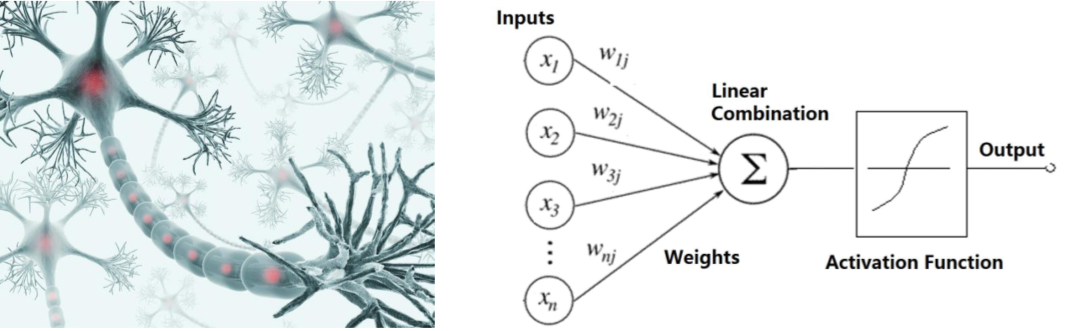以上的概念都稍显空乏，看完本节你会发现人工神经网络的的本质。

## 前馈神经网络

前馈神经网络是一种最简单的神经网络，各神经元分层排列。每个神经元只与前一层的神经元相连。接收前一层的输出，并输出给下一层．各层间没有反馈
首先我们来看一个前馈神经网络的基本结构和要素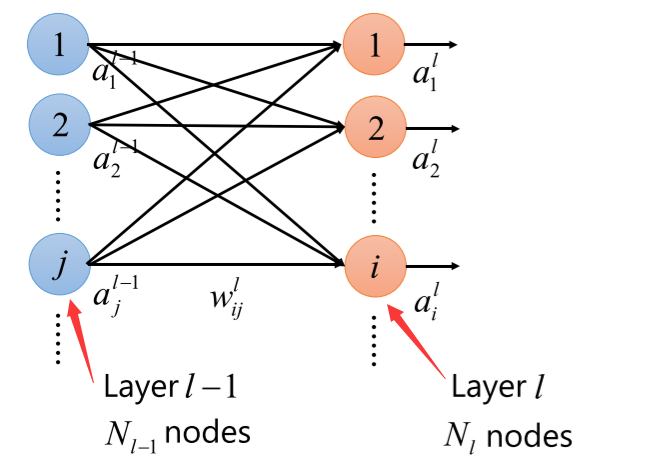上图神经网络在做的工作是，以l-1层的变量为输入，进行以w为基础的线性变换得到l层的输出
其中左边一列为上一层（l-1）右边一列为下一层（l）我们目前只关注这两层之间的计算问题
要进行线性变换，我们必须明晰权重w的表示方式如上图所示，右上角标为l，表示此时的权值为第l-1层到l层的权值，右下角标为ij表示，此权值是前一层第j个节点到下一层第i个节点的权值。那么所有的权值就构成了一个权值矩阵，如下图所示：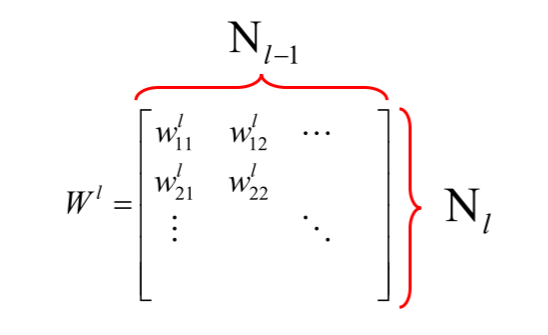列数为上一层的元素个数，行数为下一层的元素个数。但是我们发现要进行线性变换仅靠变量与权重的乘积还不够，需要添加偏置值b，以构成Σwx + b的线性组合模式
继续引入b作为偏置量，如下图所示：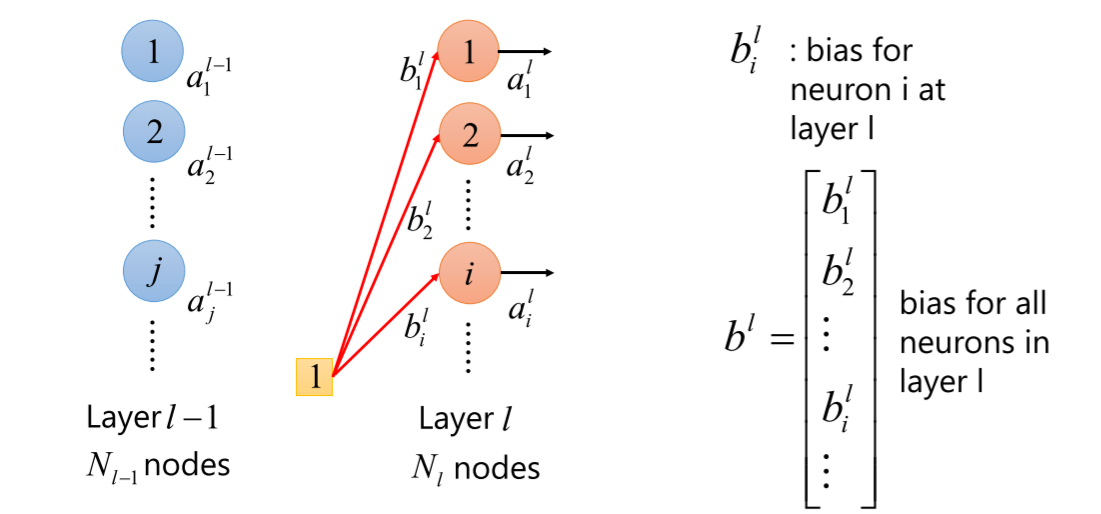对所有偏置量乘1操作即可，由图中可以看到偏置量b1~bN可以组成一个列向量，行数为l层的元素个数
接下来我们取出一个下层节点，将其作为例子，分析线性组合的结果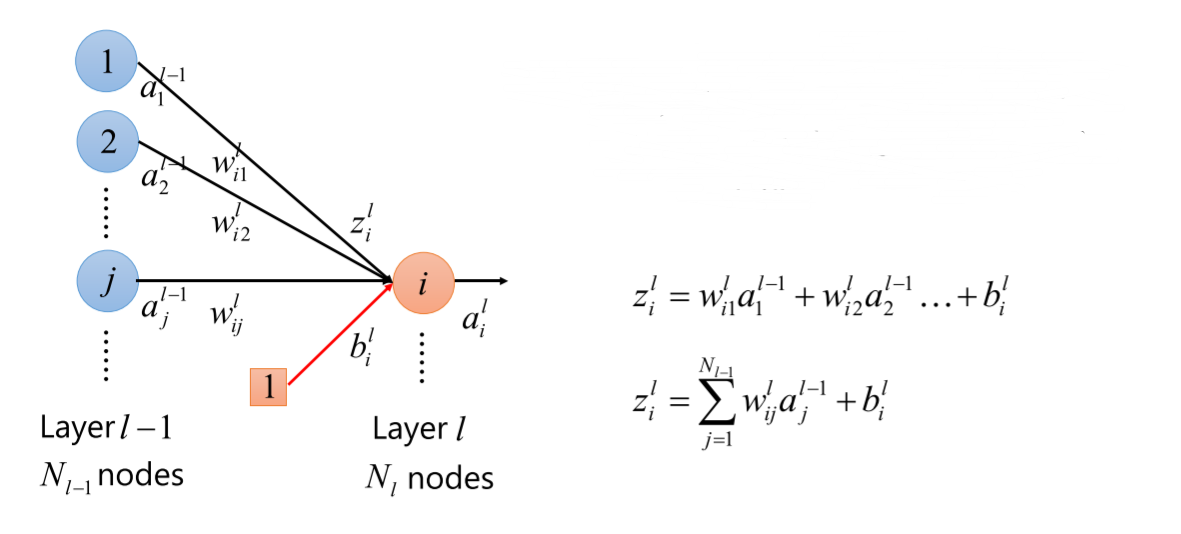可以看到下一层的某个节点的产生方式，是上一层所有节点乘以相应的权重再加偏置量得到的，注意上层节点个数和下层节点个数并不具有固定的关系。
接下来引深至下一层的全部元素，网络结构如下图所示：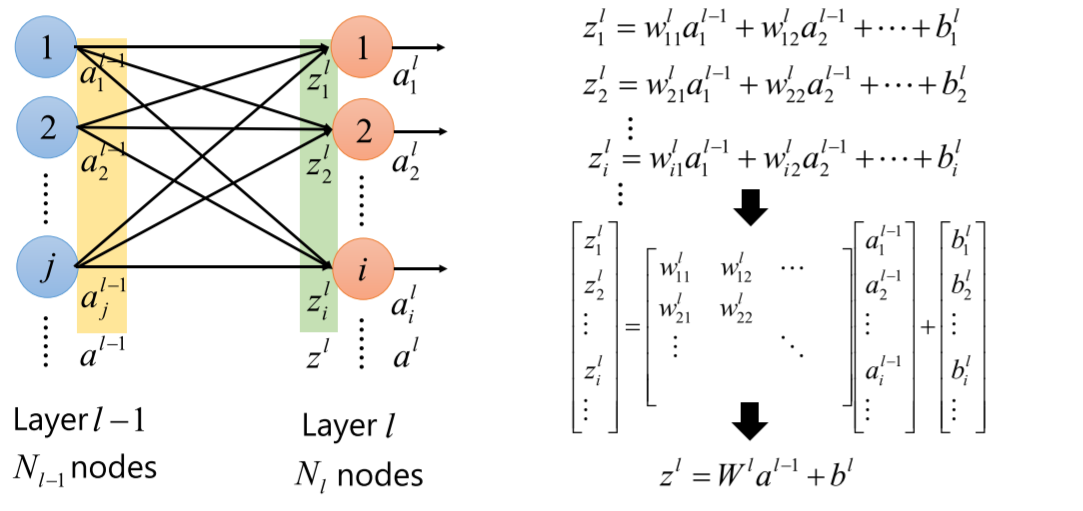计算后一层元素（列向量）的方法就十分明了了，权重矩阵乘前一层元素组成的列向量，再加上偏置量。
到了这一步，神经网络的处理依然没有结束，还需要激活函数的处理，经常使用的激活函数有sigmoid函数，不了解的同学可以参考下图形式：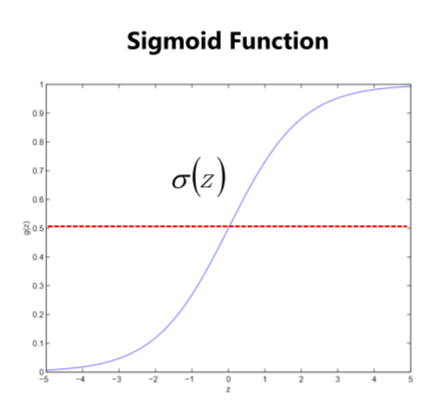sigmoid函数如下所示：sigmoid函数将一个由负无穷到正无穷区间的输入转化到0和1之间，在做分类问题时可以把0.5作为分类的标准
由此也可以看出，一个人工神经网络实际上是多个同时运行的逻辑回归。
为什么要引入激活函数？
引入激活函数，为了让中间输出多样化，能够处理更复杂的问题。
如果不使用该函数的话，每一层最后输出的都是上一层输入的线性函数，不管加多少层神经网络，我们最后的输出也只是最开始输入数据的线性组合而已。激活函数给神经元引入了非线性因素，当加入多层神经网络时，就可以让神经网络拟合任何线性函数及非线性函数，从而使得神经网络可以适用于更多的非线性问题，而不仅仅是线性问题

展开全文深度学习
• 里面包含TensorFlow和sklearn基于CIFAR-10数据集的前馈神经网络实现，以及各自的结果图片。机器学习
• Lyapunov指数是混沌动力学系统的重要不变量.用基于前馈神经网络的自适应快速学习算法计算混沌动力学系统的全部Lyapunov指数.结果表明,本算法在有限的样本点及出现外部噪声的情况下,是非常可靠的.
• 本文考虑采用平滑L0正则化（BGSL0）的批梯度方法进行训练和修剪前馈神经网络。 我们展示了为什么BGSL0可以产生稀疏的权重，这对于修剪网络。 我们证明了在温和条件下BGSL0的弱收敛和强收敛。 还获得了误差函数在训练...研究论文...